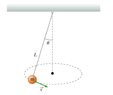# Simplify this expression for the period

crememars
Homework Statement:
A bob of mass m is suspended from a fixed point with a massless string of length L. You are to investigate the motion in which the string moves in a cone with half-angle, 𝜃. Express your answers in terms of some or all of the variables m, L, and 𝜃, and g.

v = √Lgsin𝜃tan𝜃

How long does it take the bob to make one full revolution (one complete trip around the circle)?
Relevant Equations:
T = 2πr / v
r = Lsin𝜃
hi ! I'm having a lot of trouble simplifying my expression for one of my homework questions. I know someone asked about this homework problem already, but the answers didn't really help me figure out how to simplify it.. I really have no idea what steps to take, and I've even consulted all my math notes too.

my expression for T is:

T = 2πLsinθ / √(Lgsinθtanθ)
T = 2πLsinθ * √(Lgsinθtanθ) / Lgsinθtanθ
T = 2π * √(Lgsinθtanθ) / gtanθ

[ 2π * √(Lgsinθtanθ) ] / gtanθ

2π * √( Lcosθ / g )

#### Attachments

•Screen Shot 2022-10-12 at 4.43.27 PM.png
6.7 KB · Views: 9

Homework Helper
Hi,

How about the forces that act on the bob ? Can you draw a diagram ?

##\ ##

Homework Helper
Gold Member
If you square the denominator, you can put it inside the radical. What do you get?

crememars
If you square the denominator, you can put it inside the radical. What do you get?
tried that, and it worked, thank u ! what is that rule called though? I don't remember learning it

$$\sqrt{\frac{A}{B^2}}=\frac{\sqrt{A}}{\sqrt{B^2}}=\frac{\sqrt{A}}{B}$$
•